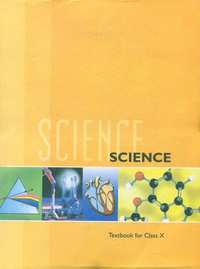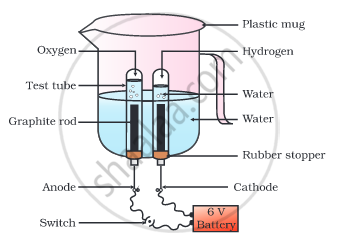Share

Books Shortlist

NCERT solutions for Class 10 Science chapter 1 - Chemical Reactions and Equations

Science Textbook for Class 10

NCERT Science Class 10Chapter 1: Chemical Reactions and Equations

Chapter 1: Chemical Reactions and Equations solutions [Page 6]

Q 1 | Page 6

Why should a magnesium ribbon be cleaned before it is burnt in air?

Q 2.1 | Page 6

Write the balanced equation for the following chemical reaction

Hydrogen + Chlorine → Hydrogen chloride

Q 2.2 | Page 6

Write the balanced equation for the following chemical reaction

Barium chloride + Aluminium sulphate → Barium sulphate + Aluminium chloride

Q 2.3 | Page 6

Write the balanced equation for the following chemical reaction

Sodium + Water → Sodium hydroxide + Hydrogen

Q 3.1 | Page 6

Write a balanced chemical equation with state symbols for the following reaction.

Solutions of barium chloride and sodium sulphate in water react to give insoluble barium sulphate and the solution of sodium chloride.

Q 3.2 | Page 6

Write a balanced chemical equation with state symbols for the following reaction.

Sodium hydroxide solution (in water) reacts with hydrochloric acid solution (in water) to produce sodium chloride solution and water.

Chapter 1: Chemical Reactions and Equations solutions [Page 10]

Q 1 | Page 10

A solution of a substance ‘X’ is used for white washing.
(i) Name the substance ‘X’ and write its formula.
(ii) Write the reaction of the substance ‘X’ named in (i) above with water.

Q 2 | Page 10

Why is the amount of gas collected in one of the test tubes in Activity 1.7 double of the amount collected in the other? Name this gas.Chapter 1: Chemical Reactions and Equations solutions [Page 13]

Q 1 | Page 13

Why does the colour of copper sulphate solution change when an iron nail is dipped in it?

Q 2 | Page 13

Give an example of a double displacement reaction other than the one given in Activity.

Q 3 | Page 13

Identify the substances that are oxidised and the substances that are reduced in the following reaction.

4Na (s) + O2 (g) → 2Na2O (s)

Q 3.2 | Page 13

Identify the substances that are oxidised and the substances that are reduced in the following reaction.

CuO (s) + H2 (g) → Cu (s) + H2O (l)

Chapter 1: Chemical Reactions and Equations solutions [Pages 14 - 16]

Q 1 | Page 14

1. Which of the statements about the reaction below are incorrect?
2PbO (s) + C (s) → 2Pb (s) + CO2 (g)
(b) Carbon dioxide is getting oxidised.
(c) Carbon is getting oxidised.
(d) Lead oxide is getting reduced.

(i) (a) and (b)
(ii) (a) and (c)
(iii) (a), (b) and (c)
(iv) all

Q 2 | Page 14

Fe2O3 + 2Al → Al2O3 + 2Fe
The above reaction is an example of a
(a) combination reaction.
(b) double displacement reaction.
(c) decomposition reaction.
(d) displacement reaction.

Q 3 | Page 15

What happens when dilute hydrochloric acid is added to iron fillings? Tick the correct answer.

1. Hydrogen gas and Iron chloride are produced.
2. Chlorine gas and Iron hydroxide are produced.
3. No reaction takes place.
4. Iron salt and water are produced.
Q 4 | Page 15

What is a balanced chemical equation? Why should a chemical equation be balanced?

Q 5.1 | Page 15

Translate the following statements into chemical equations and then balance them.

•  Hydrogen gas combines with nitrogen to form ammonia
Q 5.2 | Page 15

Translate the following statements into chemical equations and then balance them.

•  Hydrogen sulphide gas burns in air to give water and sulphur dioxide.
Q 5.3 | Page 15

Translate the following statement into chemical equation and then balance them.

• Barium chloride reacts with aluminium sulphate to give aluminium chloride and a precipitate of barium sulphate.
Q 5.4 | Page 15

Translate the following statements into chemical equations and then balance them.

• Potassium metal reacts with water to give potassium hydroxide and hydrogen gas
Q 6.1 | Page 15

Balance the chemical equation.

HNO3+Ca(OH)2Ca(NO3)2+H2O

Q 6.2 | Page 15

Balance the following chemical equation.

NaOH+H2SO4Na2SO4+H2O

Q 6.3 | Page 15

Balance the following chemical equation.

NaCl+AgNO3AgCl+NaNO3

Q 6.4 | Page 15

Balance the following chemical equation.

BaCl2 + H2SO4 → BaSO4 + HCl

Q 7.1 | Page 15

Write the balanced chemical equation for the following reaction.

Barium chloride + Potassium sulphate → Barium sulphate + Potassium chloride

Q 7.2 | Page 15

Write the balanced chemical equations for the following reaction.

Aluminium + Copper chloride → Aluminium chloride + Copper

Q 7.3 | Page 15

Write the balanced chemical equation for the following reaction.

Zinc + Silver nitrate → Zinc nitrate + Silver

Q 7.4 | Page 15

Write the balanced chemical equation for the following reaction.

Calcium hydroxide + Carbon dioxide → Calcium carbonate + Water

Q 8.1 | Page 15

Write the balanced chemical equation for the following and identify the type of reaction

Potassium bromide (aq) + Barium iodide (aq) → Potassium iodide (aq) + Barium bromide(s)

Q 8.2 | Page 15

Write the balanced chemical equation for the following and identify the type of reaction

Zinc carbonate (s) → Zinc oxide (s) + Carbon dioxide (g)

Q 8.3 | Page 15

Write the balanced chemical equation for the following and identify the type of reaction

Hydrogen (g) + Chlorine (g) → Hydrogen chloride (g)

Q 8.4 | Page 15

Write the balanced chemical equation for the following and identify the type of reaction

Magnesium (s) + Hydrochloric acid (aq) → Magnesium chloride (aq) + Hydrogen (g)

Q 9 | Page 15

What does one mean by endothermic reaction? Give example.

Q 9 | Page 15

What does one mean by exothermic reaction? Give example.

Q 10 | Page 15

Why is respiration considered an exothermic reaction? Explain.

Q 11 | Page 15

Why are decomposition reactions called the opposite of combination reactions? Write equations for these reactions.

Q 12 | Page 16

Write one equation each for decomposition reactions where energy is supplied in the form of heat, light or electricity.

Q 13 | Page 16

What is the difference between displacement and double displacement reactions? Write equations for these reactions.

Q 14 | Page 16

In the refining of silver, the recovery of silver from silver nitrate solution involved displacement by copper metal. Write down the reaction involved.

Q 15 | Page 16

What do you mean by a precipitation reaction? Explain by giving examples.

Q 17 | Page 16

A shiny brown-coloured element ‘X’ on heating in air becomes black in colour. Name the element ‘X’ and the black coloured compound formed

Q 19 | Page 16

Oil and Fat containing food items are flushed with Nitrogen. Why?

Q 20.1 | Page 16

Explain the terms Corrosion

Q 20.2 | Page 16

Explain the term Rancidity.

Chapter 1: Chemical Reactions and Equations

NCERT Science Class 10NCERT solutions for Class 10 Science chapter 1 - Chemical Reactions and Equations

NCERT solutions for Class 10 Science chapter 1 (Chemical Reactions and Equations) include all questions with solution and detail explanation. This will clear students doubts about any question and improve application skills while preparing for board exams. The detailed, step-by-step solutions will help you understand the concepts better and clear your confusions, if any. Shaalaa.com has the CBSE Science Textbook for Class 10 solutions in a manner that help students grasp basic concepts better and faster.

Further, we at shaalaa.com are providing such solutions so that students can prepare for written exams. NCERT textbook solutions can be a core help for self-study and acts as a perfect self-help guidance for students.

Concepts covered in Class 10 Science chapter 1 Chemical Reactions and Equations are Types of Chemical Reactions - Double Displacement, Types of Chemical Reactions - Displacement, Types of Chemical Reactions - Decomposition, Types of Chemical Reactions - Combination, Effects of Oxidation Reactions in Everyday Life - Rancidity, Effects of Oxidation Reactions in Everyday Life - Corrosion, Balanced Chemical Equation, Chemical Equation, Types of Chemical Reactions - Neutralization, Types of Chemical Reactions - Oxidation and Reduction.

Using NCERT Class 10 solutions Chemical Reactions and Equations exercise by students are an easy way to prepare for the exams, as they involve solutions arranged chapter-wise also page wise. The questions involved in NCERT Solutions are important questions that can be asked in the final exam. Maximum students of CBSE Class 10 prefer NCERT Textbook Solutions to score more in exam.

Get the free view of chapter 1 Chemical Reactions and Equations Class 10 extra questions for Science and can use shaalaa.com to keep it handy for your exam preparation

S